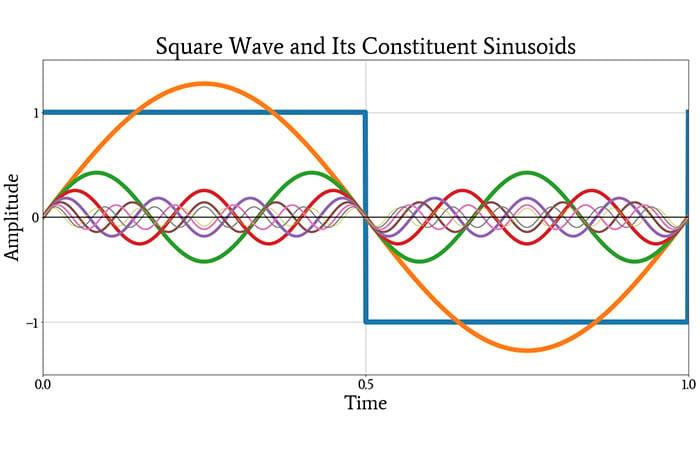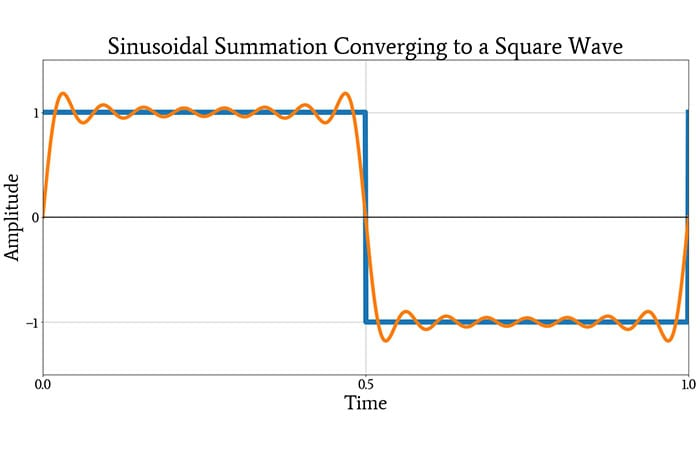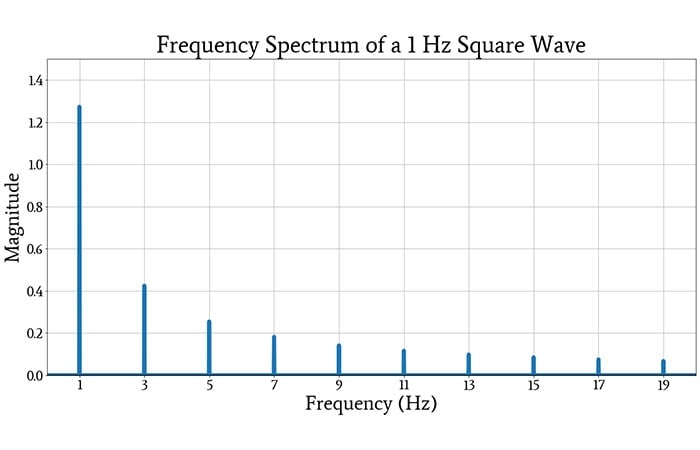Technical Article

# What Is the Fourier Transform?

July 30, 2020 by Robert Keim

## This article gives you essential information on a mathematical technique that plays an absolutely fundamental role in system design and signal processing.

Named after French mathematician Joseph Fourier, the Fourier transform is a mathematical procedure that allows us to determine the frequency content of a function. For electrical engineers, the Fourier transform is typically applied to functions of time that we call signals.

### Sinusoidal Decomposition

A plot of voltage or current vs. time, as we would see on an oscilloscope display, is an intuitive representation of signal behavior. It is not, however, the only useful representation.

In many cases—for example, in the design of RF systems—we are interested primarily in the periodic behavior of signals. More specifically, we are interested in understanding a signal with respect to sinusoidal periodicity, because sinusoids are the unique mathematical expression of “pure” frequency.

The Fourier transform reveals a signal’s elemental periodicity by decomposing the signal into its constituent sinusoidal frequencies and identifying the magnitudes and phases of these constituent frequencies.

The word “decomposing” is crucial here. The Fourier transform teaches us to think about a time-domain signal as a waveform that is composed of underlying sinusoidal waveforms with various magnitudes and phases.

A square wave, for example, can be decomposed into an infinite series of sinusoids with amplitudes that steadily decrease and frequencies that steadily increase. The exact series, for an AC-coupled square wave of period T and amplitude A, can be written as follows:

$f_{square}(t)=\frac{4A}{\pi}\sum_{k\in{\{1,3,5,...\}}}\frac{1}{k}\sin\left(\frac{2\pi kt}{T}\right)$

We can convert this into the following form, which is a bit more intuitive:

$f_{square}(t)=\frac{4A}{\pi}\left(\sin(2\pi ft)+\frac{1}{3}\sin(6\pi ft)+\frac{1}{5}\sin(10\pi ft)+\ ...\right)$

where f is the frequency, in hertz, of the square wave.

The following plot shows the original square wave, in blue, and the first eight sinusoids in the infinite series.

#####After looking at this plot, you might still be a bit skeptical that these sinusoids can be combined into a square wave. The next plot will convince you, though. It shows the original square wave and the waveform produced by adding all of the constituent sinusoids shown above.### Functions of Time and Frequency

When we calculate a Fourier transform, we begin with a function of time, f(t), and through mathematical decomposition, we produce a function of frequency, F(ω). (We typically use angular frequency in theoretical discussions of the Fourier transform.)

Evaluating F(ω) at some specific angular frequency, say 100 rad/s, gives us the magnitude and phase of the sinusoidal component of f(t) that has a frequency of 100 rad/s. If f(t) does not have a sinusoidal component at 100 rad/s, the magnitude will be zero.

You might be wondering how one function, F(ω), can report both magnitude and phase. The Fourier transform produces a complex-valued function, meaning that the transform itself is neither the magnitude of the frequency components in f(t) nor the phase of these components. As with any complex number, we must perform additional calculations to extract the magnitude or the phase.

The concept of a complex-valued transform is somewhat more intuitive when we’re working with a discrete Fourier transform, rather than a “standard” transform in which we begin with a symbolic function of time and end with a symbolic function of frequency.

The discrete Fourier transform operates on a sequence of numerical values, and it produces a sequence of Fourier coefficients. These coefficients are typical complex numbers (i.e., they have the form a + jb), and we usually use the magnitude of these complex numbers, calculated as √(a2+b2), when analyzing the frequency content of a signal.

### Plotting the Fourier Transform

Plots of frequency content are extremely common in datasheets, test reports, textbooks, and so forth. We often refer to a plot of magnitude vs. frequency as a spectrum—for example, “let’s take a look at the spectrum of the signal” means “let’s take a look at some sort of visual representation of the magnitude information in the Fourier transform.”

The following plot shows the spectrum of an AC-coupled square wave with an amplitude of 1 and a frequency of 1 Hz.If you compare the plotted amplitudes of the frequency “spikes” to the amplitudes of the corresponding sinusoidal components in the infinite series discussed above, you will see that they are consistent.

### Calculating the Fourier Transform

We’re almost at the end of this article, and I still haven’t told you how we actually generate the Fourier transform of a mathematically defined signal.

To be honest, I don’t see a need to thoroughly explore mathematical details in an introductory article: frequency-domain analysis nowadays is dominated by user-friendly, software-based techniques, and engineers don’t spend much time converting symbolic time-domain expressions into symbolic frequency-domain expressions.

Nevertheless, with something as important as the Fourier transform, it’s good to at least be aware of the underlying math. So, without further ado, this is how we convert f(t) into F(ω):

$F(\omega ) = \int\limits_{ - \infty }^{ + \infty } {f(t){e^{ - j\omega t}}dt}$

### Conclusion

I hope that this article has provided a clear, intuitive explanation of what the Fourier transform is and how it gives us additional insight into the nature of a signal.

The Fourier transform is just the beginning of an expansive array of related topics; if you’d like to learn more, take a look at the articles listed below.

1 Comment• B
Bernie Hutchins August 02, 2020

Robert -

What you title here as “Fourier Transform” (FT), and follow with a square-wave example, is much more properly called a “Fourier Series” (FS) as you really MUST know!  Only with your very last equation (the integral - written down without elaboration) do you present anything properly (directly) called a FT.

One may choose to use the term FT to ENCOMPASS the full, beautifully-symmetric, set of FOUR transform PAIRS [ 1 - Continuous-Time FT (CTFT) , 2 - FS,  3 - Discrete-Time FT (DTFT), and 4 - Discrete FT (DFT = FFT) ].)  See http://electronotes.netfirms.com/AN410.pdf

While general inclusion within the Fourier “Trademark” offers some overall insight, it does not PERMIT the choice of the wrong specific terminology – let alone the attempted (illogical/inappropriate) use of one of the four alternatives.

For another example, you cite a “discrete Fourier transform” which is NOT what everyone else considers to be a DFT (discrete in time and in frequency, and periodic in both domains).  Rather it is a DTFT; continuous – like the “frequency response” of a digital filter’s discrete impulse response.

- Bernie

Like.